```
Digital  interview questions Page 1   Page 2

Page 3  Page 4 Page 5
```

What is the decimal equivalent of the largest binary integer that can be obtained with (a) 16 bits and (b) 24 bits?

(a) 216-1 = 65,535. (b) 224-1 = 16,777,215.

Convert the following binary numbers to decimal: (a) 1010010, (b) 10101010.101, and (c) 10100110?.

(a) 1010010 = 26 + 24 + 21 = 82. <br>

(b) 10101010.101 = 27 + 25 + 23 + 21 + 2-1 + 2-3 = 170.625. <br>

(c) 10100110 = 27 + 25 + 22 + 21 = 166.  <br>

A computer represents information in groups of 48 bits. How many different integers can be represented in (a) binary, (b) BCD, and (c) 8-bit ASCII, using all 48 bits in a word?

(a)   248 256×1012 (b) 1048/4 =1012 (c) 1048/8 =106.

Construct a timing diagram for the circuit shown below, assuming inputs ABC are all low from time 0 to 10, ABC=LLH from time 10 to 20 (where L denotes low, H denotes high), ABC=LHL from time 20 to 30, ABC=LHH from time 30 to 40, ABC=HLL from time 40 to 50, ABC=HLH from time 50 to 60, ABC=HHL from time 60 to 70, ABC=HHH from time 70 to 80 ?Draw a logic diagram that directly corresponds to each of the expressions shown below. (a) AB′ + ACD′ + ABD + ABCD′ (b) B′ + ACD′ (c) (A+ B+ C + D′)(A + B + C+ D)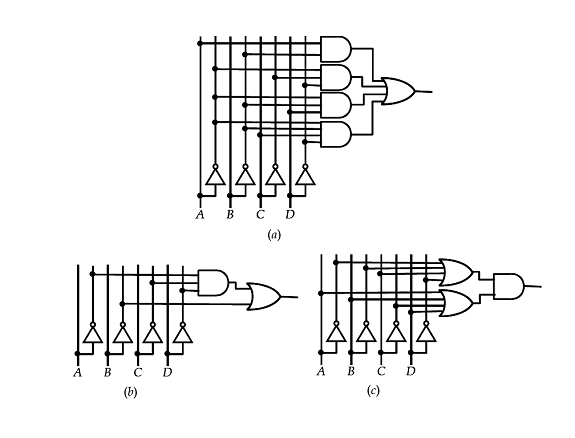Simplify the following Boolean expressions using only the Boolean theorems in the notes to a minimum number of literals?

a. ABC + ABC’ + A’B

b. (A + B)’(A’ + B’)

c. (A + B’ + AB’)(AB + A’C + BC)

d. ((A + B)C’+AB’)B’D

a. ABC + ABC’ + A’B = AB(C + C’) + A’B (T8)

= AB + A’B (T5)(T1’) = B(A + A’) (T6’)(T8) = B (T5)(T1’)

b. (A + B)’(A’ + B’)

= A’B’(A’ + B’) (T13) = A’B’ + A’B’ (T8)(T6’)(T3’) = A’B’ (T3)

c. (A + B’ + AB’)(AB + A’C + BC)

= (A + AB’ + B’ + AB’)(AB + A’C) (T3)(T6)(T11) = (A + B’)(AB + A’C) (T9) = AB + A’B’C (T8)(T5’)(T2’)

d. ((A + B)C’+AB’)B’D

= (B’A + BC’ + AC’)B’D (T6’)(T8)(T6) = (B’A + BC’)B’D (T11) = AB’D (T6’)(T5’)(T2’)

Find an equivalent NOR circuit for the following NAND circuit. ?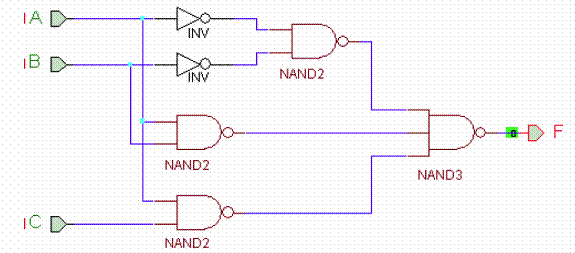This circuit is F=A’B’+AB+AC = (A+B’)(A’+B+C). After entering both circuits, the output is, indeed, zero everywhere. The XOR gate is zero when the inputs are equal and since these circuits are equivalent, the output is always zero.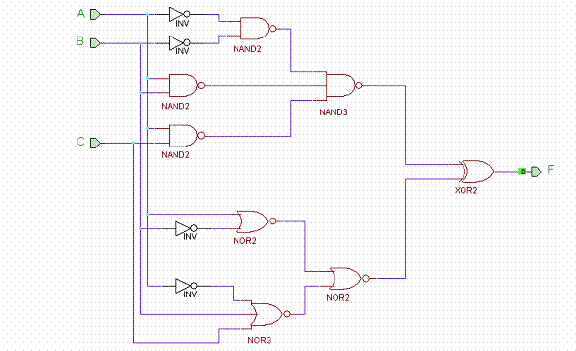You work for the mega-design company Uetni. Due to the recent economic downturn, all circuit designs are being reevaluated to reduce costs. You are given the task of reducing cost in the following circuit. Assuming that all gates cost \$0.10 per input (an inverter is \$0.10, a 2-input gate is \$0.20, etc.) find the minimum cost circuit for the following using only inverters, AND/OR gates.?From the diagram we find that F=AD’+A’C’+A’C’D’+A’B’C. First, let’s see what the minimal equations are in both SOP and POS forms:Which of the following CMOS circuits would have the shortest delay? The longest? Assume that the sizes of the CMOS transistors in all gates are the same and that the load on the output is equivalent to that of another inverter. ?For each parallel inverter driving the same load, the speedup is about 2x. For A we have a delay of 2 units. In B, we have 0.5 units for the first group and 1 unit for the second group for a total of 1.5 units. For C, we have 1 unit for the first group for the load is twice as much to drive two inverters as one; the second group has 0.5 unit delay and the last inverter has 1 units for a total of 2.5 units. Circuit D is the best with the first group of inverters having 0.5 units delay and the second group having 0.5 units delay for a total of 1 unit delay.<br><br> Circuit D is the fastest, and circuit C is the slowest!

How many transistors would be required for Following Circuit?The circuit has five 2-input NAND gates and one 3-input NAND gate. Each 2-input NAND gate takes four transistors and each 3-input NAND gates needs six. So (5*4) + 6 = 26 transistors

Find a circuit using the minimum number of 4-input LUTs that implements the three logic functions, below: ?

X = (A + DF’)(BC + BC’E)

Y = (B’+C’E’) + (A’D + AD’ + DF)

Z = AB(C + E)(D’ + A’F)

If we are a little clever and let U = (BC+BC’E), then we can rewrite the three expressions as

X = (A + DF’) U

Y =(U + (A’D + AD’ + DF)

Z = AU(D’ + A’F) [ for BC+BC’E = B(C+E)]

Each of these expressions is only a function of four inputs (A, D, F and U) so we can use one LUT4 for each plus one more to generate U:Construct a 10-to-1 line multiplexer with three 4-to-1 line multiplexers. The multiplexers should be interconnected and inputs labeled so that the selection codes 0000 through 1001 can be directly applied to the multiplexer selections inputs without added logic ?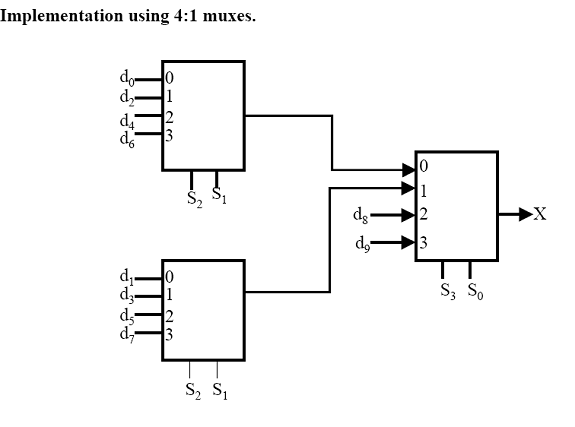In elementary school, we learned to do arithmetic with positive and negative numbers using sign-magnitude arithmetic. Using this method, when performing the subtraction operation AB on two non-negative numbers, we subtract the smaller number from the larger and make the sign of the result positive if A>B and make the sign negative if A<B. Draw a schematic that implements this method of subtracting two 4 bit values in sign-magnitude representation. You may assume that the two input values are non-negative (so bit 3 of both input values will be zero), but your circuit should produce the correct sign for the result. Your circuit may use the following building blocks in addition to simple gates: a 3 bit comparison circuit, a 3 bit subtraction circuit, and one or more 2:1 multiplexors.?

First, see if A<B. If it is, we will get a negative number that equals –(B-A). If A>B then we get a positive number that is A-B. Directly implementing this is what the diagram does.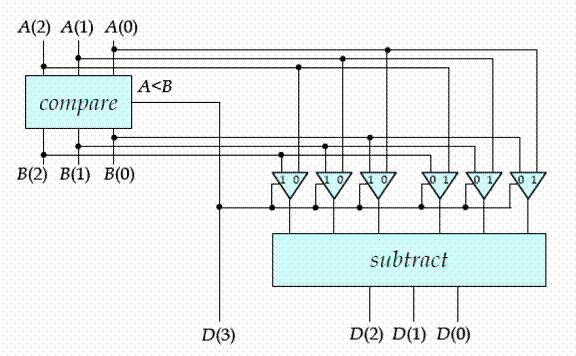Assume that each flip-flop has a setup time of 2 ns, a hold time of 3 ns and a clock-to-output delay of 5 ns. Further assume that each gate has a delay of 2 ns except each inverter has a delay of 1 ns. What is the maximum clock frequency that you can clock the following circuits. Also discuss what constraints are placed on the inputs

Assume that each flip-flop has a setup time of 2 ns, a hold time of 3 ns and a clock-to-output delay of 5 ns. Further assume that each gate has a delay of 2 ns except each inverter has a delay of 1 ns. What is the maximum clock frequency that you can clock the following circuits. Also discuss what constraints are placed on the inputs. ?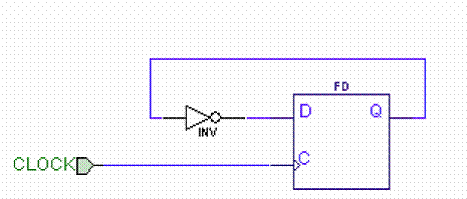a. From clock, we wait 5 ns for Q to change so we meet the hold time (3 ns) with no problems. Setup time is 2 ns and we need to wait at least 6 ns (5+1 ns) for the output to show up at the input, D. Hence, we can clock this every 6+2 ns = 8 ns or 125 MHz.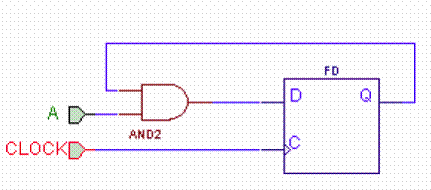b. Again, hold is not a problem but the delay is now 7 ns from clock to the Q output showing up at D. Adding setup, 7+2 = 9 ns, we can clock at about 111 MHz. Input A must be stable 4 ns before clock (2ns setup plus the 2 ns for the AND gate delay.c. Here, the worst case delay is from the clock to the output of the first flip-flop (5 ns), through the AND gate (2 ns) and to the D input (2 ns setup). There is no hold time problem with this circuit since all delays are more than 3 ns. Input B must be stable at least 4 ns before clock to meet the setup time. Hence, we can clock this circuit no faster than about 111 MHz.

Consider the sequential circuit shown below. Assume that the flip flops have a setup time of 2 ns, a hold time of 1 ns and a propagation delay between 1 and 3 ns. Also assume that the maximum clock skew is 1 ns and that all the gates have a propagation delay between .5 and 2 ns. What is the shortest clock period for which we can be certain that there are no violations of setup times, assuming no changes at the input X? Is the circuit subject to hold time violations? If so, what would you do to eliminate the hold time violations? If the clock goes high at time 0, during what time period must X be stable to ensure that there are no violations of setup and hold times? During what time period is it possible for the output to be changing. If the clock could change anytime between t=-1 ns and t=+1 ns, how do the last two answers change?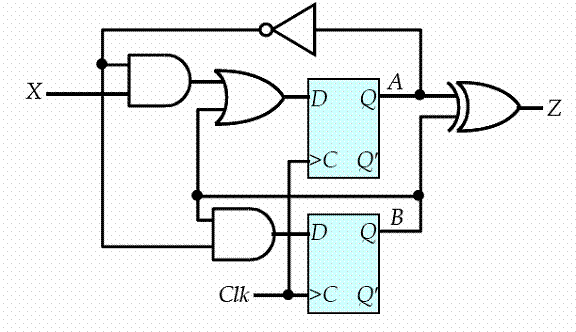First, let’s look at hold time: Hold time violations occur when we clock a flip-flop and this causes the output to propagate to a flip-flop input before the hold time is satisfied. Hence, look for the shortest path from Q to D. This occurs from QB to DB and DB. With a clock skew of 1 ns, however, the worst case is when that causes QB to change before flip-flop A ever gets clocked! (There can be no clock skew when the output goes to the input of the same flip-flop.) Hence, the worst case hold time is from QB to DA through the one OR gate. The total delay could be as fast as 1 ns flip flop propagation delay + 0.5 ns OR gate delay = 1.5 ns while we might need to hold the value for 1 ns clock skew + 1 ns hold time = 2 ns. Hence, we have a problem and to fix it we will add two inverters (0.5 ns delay on both) to the feedback of QB to lengthen this path to 2.5 ns which is greater than the hold time we need (2 ns). All other feedback paths are at least 2 ns so we are OK on them. Now, the setup time analysis will tell us how fast we can clock the circuit. We see that the longest path from Q to D is from QA to DA. Since this is from the Q to D of the same flip-flop, let’s also look at the longest path between the flip-flops to be sure clock skew doesn’t affect this analysis.

From Qto DAA A: 3 ns flip flop propagation delay + 3*2 ns comb. circuit delay + 2 ns setup time = 11 ns. From QB to D: 3 ns flip flop propagation delay + 3*2 ns comb. circuit delay (don’t forget our added inverters!) + 1 ns clock skew + 2 ns setup time = 12 ns. Using the maximum values for the propagation delays we found that the period must be at least 3+(3*2)+1+2=12 ns. If the clock changes at t=0, then X must be stable starting at t=-6 and continuing until t=0. If the clock can change anytime within 1 ns of t=0, then X must be stable starting a t= -7 and continuing until time t =+1. The output Z can change anytime between t= +1.5 ns and t= +5ns, if the clock changes at t=0, but if the clock can change anytime in the interval between –1 and +1, then the output can change anytime between +.5 and +6.

The following circuit is used as a synchronizer for the traffic sensor used on the traffic controller designed in class. Assume the traffic controller is clocked with a 10 Hz clock. We expect the sensor to be activated about once a minute during the day.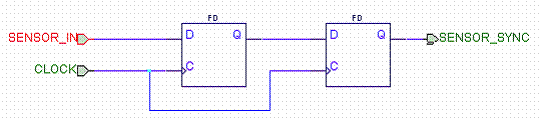How fast should we clock this synchronizer? Since it is a synchronizer, we must clock it at the same speed as the state machine we’re feeding: 10 Hz. Any other clock rate and we’re not synchronized!

b. Shouldn’t we have a reset on these flip-flops? Why or why not?

If we do not reset both flip-flops and either flip-flop starts at a logic 1 when the SENSOR_IN is not, then we might get a false-positive on the sensor in put and make the light change when no cars are around. This is only a problem at startup of the system, however. After this initial startup period, the SENSOR_IN will be a logic 0 and will, effectively, reset this synchronizer for us. If we don’t care what happens when we turn the system on, we can ignore reset, but we probably should have a reset to be robust. The only problem is then we must ensure that the reset timing is such that we don’t have a possible metastability issue.

c. Assuming we clock this synchronizer at 10 Hz, can we assume that since the input, SENSOR_IN, only changes once a minute (1/60 Hz) that it will never fail (i.e. latch a metastable state)? No. The probability is low, but nothing is guaranteed! If we happen to have a car arrive on the sensor just as this circuit is getting clocked, we will violate a setup and/or hold time and have the possibility of a metastable state being latched.

d. Assuming we cannot change the clock frequency or rate of input changes, is there any way to reduce the probability that we will have a failure of the synchronizer? If we have flip-flops with shorter setup times this will give us a smaller chance of violating it and will also give us a longer resolution time. Other than that, there is nothing we can do.

Verilog FAQ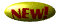Synthesis FAQ

Digital FAQ

Timing FAQ

ASIC FAQCmos FAQ

Misc FAQ

Home